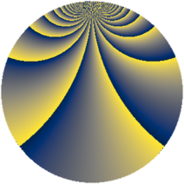# Properties

 Label 900.3.bpLevel $900$ Weight $3$ Character orbit 900.bp Rep. character $\chi_{900}(31,\cdot)$ Character field $\Q(\zeta_{30})$ Dimension $2848$ Sturm bound $540$

# Related objects

## Defining parameters

 Level: $$N$$ $$=$$ $$900 = 2^{2} \cdot 3^{2} \cdot 5^{2}$$ Weight: $$k$$ $$=$$ $$3$$ Character orbit: $$[\chi]$$ $$=$$ 900.bp (of order $$30$$ and degree $$8$$) Character conductor: $$\operatorname{cond}(\chi)$$ $$=$$ $$900$$ Character field: $$\Q(\zeta_{30})$$ Sturm bound: $$540$$

## Dimensions

The following table gives the dimensions of various subspaces of $$M_{3}(900, [\chi])$$.

Total New Old
Modular forms 2912 2912 0
Cusp forms 2848 2848 0
Eisenstein series 64 64 0

## Trace form

 $$2848q - 3q^{2} - 3q^{4} - 8q^{5} + 2q^{6} - 12q^{8} - 12q^{9} + O(q^{10})$$ $$2848q - 3q^{2} - 3q^{4} - 8q^{5} + 2q^{6} - 12q^{8} - 12q^{9} - 24q^{10} + 12q^{12} - 6q^{13} - 27q^{14} - 3q^{16} - 24q^{17} - 52q^{18} - 57q^{20} - 66q^{21} + 13q^{22} - 122q^{24} - 8q^{25} - 48q^{26} - 108q^{28} - 6q^{29} - 86q^{30} - 68q^{32} + 24q^{33} + 5q^{34} + 112q^{36} - 24q^{37} - 389q^{38} + 21q^{40} - 6q^{41} - 524q^{42} + 52q^{44} - 42q^{45} + 4q^{46} + 205q^{48} + 9280q^{49} + 207q^{50} - 67q^{52} - 312q^{53} - 193q^{54} + 144q^{56} - 136q^{57} - 19q^{58} + 1039q^{60} - 6q^{61} - 176q^{62} - 12q^{64} - 156q^{65} + 526q^{66} - 444q^{68} + 264q^{69} + 138q^{70} + 200q^{72} - 24q^{73} + 210q^{74} - 40q^{76} - 594q^{77} - 836q^{78} + 18q^{80} - 492q^{81} - 48q^{82} + 159q^{84} + 42q^{85} + 273q^{86} - 155q^{88} - 24q^{89} - 155q^{90} - 53q^{92} + 676q^{93} - 167q^{94} - 223q^{96} - 6q^{97} - 1650q^{98} + O(q^{100})$$

## Decomposition of $$S_{3}^{\mathrm{new}}(900, [\chi])$$ into newform subspaces

The newforms in this space have not yet been added to the LMFDB.